# HC Verma Solutions Class 11 Chapter 2 Physics and Mathematics

HC Verma Solutions Class 11 Chapter 2 – Physics and Mathematics are available for students who are looking to solve questions from the second chapter of HC Verma book. The solutions provided here are aimed at providing the best answers for class 11 physics students. The chapter mainly deals with mathematical formulas that are integrated with solving for different concepts in Physics. In this chapter, students further learn more in detail about different mathematical topics such as vectors, displacement vectors, resultant vectors, components and addition of vectors.

One of the more interesting concepts that this chapter teaches us, is using vectors for solving different problems related to certain shapes such as polygons and hexagons. Thus, this chapter is useful in teaching students about the different concepts of Physics and how we can use mathematical formulas to solve the different problems. Thus, a student needs to be prepared to solve different problems in the subject of Physics with the help of mathematics.

Download All the Solutions As PDF: HC Verma Solutions Chapter 2 PDF

In chapter 2 students will learn about topics like;

1. Vectors and Scalars
2. Equality of Vectors
4. Multiplication of a Vector by a Number
5. Subtraction of Vectors
6. Resolution of Vectors
7. Dot Product or Scalar Product of Two Vectors
8. Cross Product or Vector Product of Two Vectors
9. Differential Calculus as Rate Measurer
10. Maxima and Minima
11. Integral Calculus
12. Significant Digits
13. Significant Digits in Calculations
14. Errors in Measurement

## Class 11 Important Questions In Chapter 2

• When we say “direction of zero vector” does it have any physical
significance? Give your reasons in terms of velocity, force etc.
• Can a unit vector be formed by using or adding three unit vectors?
What will be your answer if two unit vectors are along the coordinate axes?
• A vector has which main component:
(a) always greater than its magnitude
(b) always less than its magnitude
(c) always equal to its magnitude
(d) none of these.
• Is it possible to have physical quantities with direction and magnitude that are not vectors?
• The radius of a circle is given as 2.12 cm. How can its area be represented?
(a) 14 cm2 (b) 14.1 cm2 (c) 14.11 cm2 (d) 14.1124 cm2.

## HC Verma Solutions Vol 1 Physics and Mathematics Chapter 2

Question 1: A vector A makes an angle of 20o and vector B makes an angle of 110 o with the x-axis. The magnitudes of these vectors are 3 m and 4 m respectively. Find the resultant.

Solution: The angle between two vectors, $\vec{A}$ and $\vec{B}$ is 90 o.

Magnitude of $\vec{A}$ and $\vec{B}$:

$|\vec{A}|=3$ and $|\vec{B}|=4$

The resultant vector, say $\vec{R}$ = $\sqrt{A^2+B^2+2AB\ cos \theta}$ = 5 m

Let angle $\beta$ be the angle between $\vec{A}$ and $\vec{R}$

$\beta = tan^{-1}(\frac{4 sin90^o}{3+4cos90^o})=tan^{-1}(4/3)=53^o$

The angle between resultant vector and x axis = 53 o + 20 o = 73 o.

Question 2: Let A and B be the two vectors of magnitude 10 unit each. If they are inclined to the x-axis at angles 30 o and 60 o respectively. Find the resultant.

Solution:

Let A and B be the two vectors of magnitude 10 unit each. (Given)

=> $|\vec{A}|= |\vec{B}|$ = 10 unit

Let θ be the angle between vectors A and B, and β be the angle between vector A and resultant vector.

=> θ = 30o

And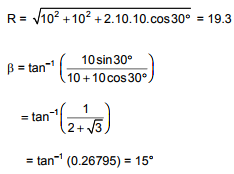Therefore, resultant vector with the x-axis = 15 o + 30 o = 45 o

Question 3: Add vectors A, B and C each having magnitude of 100 unit and inclined to the x-axis at angles 45 o, 135 o and 315 o respectively.

Solution:

Let A , B and C are three vectors of magnitude 100 unit each. (Given)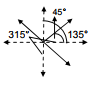Find x components of vectors, A, B and C

x-components of vector A = 100 cos 45 o = 100/ √2 unit

x-components of vector B = 100 cos 135 o = -100/ √2 unit

x-components of vector C = 100 cos 315o = 100/ √2 unit

So, resultant x-component = (100/ √2 – 100/ √2 + 100/ √2)unit = 100/ √2 unit

Find y components of vectors, A, B and C

y-components of vector A = 100 sin 45 o = 100/ √2 unit

y -components of vector B = 100 sin 135 o = 100/ √2 unit

y -components of vector C = 100 sin 315o = -100/ √2 unit

So, resultant y-component = (100/ √2 + 100/ √2 – 100/ √2)unit = 100/ √2 unit

Now,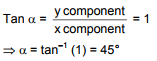The resultant is 100 unit at 45o with x-axis.

Question 4: Let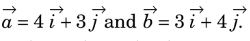Find the magnitudes of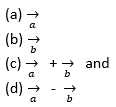Solution: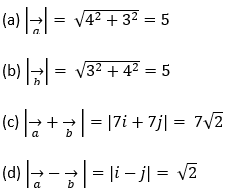Question 5: Refer to the given figure. Find (a) the magnitude, (b) x and y components and (c) the angle with the x-axis of the resultant of , and .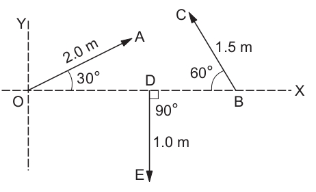Solution:

x component of Vector OA = 2 cos 30 = √3

x component of vector BC = 1.5 cos 120 = -1.5/2 = -0.75

x component of vector DE = 1 cos 270 = 1 × 0 = 0

Resultant of x component = (√3 – 0.75 + 0) m = (1.73 -0.75) m = 0.98 m

y component of Vector OA = 2 sin 30 = 1

y component of vector BC = 1.5 sin 120 = 1.3

y component of vector DE = 1 sin 270 = -1

Resultant of y component = (1 + 1.3 – 1) m = 1.3 m

Now,

Total resultant of x and y component = √[(1.3)²+(0.98)²] = 1.6 m

Let A be the angle between resultant and x-axis, then

tan A = (y component/x component) = 1.32

A = tan⁻¹(1.32)

So, the resultant is 1.6 m and tan⁻¹(1.32) with positive x-axis.

Question 6: Two vectors have magnitudes 3 unit and 4 unit respectively. What should be the angle between them if the magnitude of the resultant is (a) 1 unit, (b) 5 unit and (c) 7 unit.

Solution: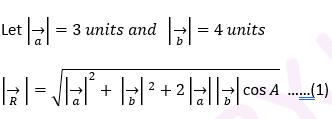(a) When resultant magnitude = 1 unit

Using (1),

1 = √[32 + 42 + 2x3x4 cos A]

24 cos A + 25 = 1

cos A = -1

Or A = 180o

(b) When resultant magnitude = 5 unit

Using (1),

52 = √[32 + 42 + 2x3x4 cos A]

25 = 25 + 24 cos A

24 cos A = 0

or A = 90o

(c) When resultant magnitude = 7 unit

Using (1),

72 = √[32 + 42 + 2x3x4 cos A]

24 = 24 cos A

cos A = 1

or A = 0o

Question 7: A spy report about a suspected car reads as follows. “The car moved 2.00 km towards east, made a perpendicular left turn, ran for 500 m, made a perpendicular right turn, ran for 4.00 km and stopped”. Find the displacement of the car.

Solution:

First convert all distance in same unit.

2 km, 500 m = 0.5 km and 4 km

In the figure,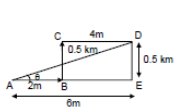vector AD = 2i + 0.5j + 4k

Using Pythagoras Theorem ,

AD = √(AE² + DE²) = 6.02 km

Now,

tan θ = (DE/AE) = 1/12

θ = tan⁻¹(1/12)

The displacement of the car along the distance tan⁻1(1/12) with positive x-axis is 6.02 km.

Question 8: A carrom board (4 ft x 4 ft square) has the queen at the centre. The queen, hit by the sticker moves to the front edge, rebounds and goes in the hole behind the striking line. Find the magnitude of displacement of the queen

(a) from the centre to the front edge

(b) from the front edge to the hole and

(c) from the centre to the hole.

Solution: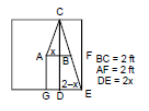In triangle ABC,

tan θ = x/2 …. (1)

In triangle DCE,

tan θ = (2-x)/4 …(2)

Equating (1) and (2), we get

x/2 = (2-x)/4

2x = 2-x

3x = 2

x = 2/3

The value of x is 2/3 ft

(a) In triangle ABC

AC = √(AB² + BC²) = (2√10)/3 ft.

[Using Pythagoras Theorem]

(b) In triangle CDE,

DE = 1 – (2/3) = 4/3 ft.

and CD = 4ft.

Now, find CE using again Pythagoras Theorem

CE = √(CD² + DE²) = (4√10)/3

CE = (4√10)/3 ft.

(c) From triangle AGE,

AE = √(AG² + GE² = 2√2 [(by Pythagoras Theorem)

Length of AE = 2√2 ft.

Question 9: A mosquito net over a 7 ft x 4 ft bed is 3 ft high. The net has a hole at one corner of the bed through which a mosquito enters the net. It flies and sit at the diagonally opposite upper corner of the net.

(a) Find the magnitude of the displacement of the mosquito.

(b) Taking the hole as the origin, the length of the bed as the x-axis, its width as the y-axis, and vertically up as the z-axis, write the components of the displacement vector.

Solution:

Write the displacement vector using given information’s.

Let vector r be the displacement vector,

Vector r = 7i + 4j + 3k

(a) Magnitude of displacement = √(7² + 4² + 3²) = √(49 + 16 +9) = √(74) ft.

(b) Components of the displacement vector r are 7 ft. , 4 ft. , and 3 ft. respectively.

Question 10: Suppose is a vector of magnitude 4.5 unit due north. What is the vector (a) 3 (b) – 4 ?

Solution:

Given: Vector a is a vector of magnitude 4.5 unit due north.

Here vector a = 4.5

(a) 3a = 3 x 4.5 =13.5

Vector (3a) is along north with magnitude 13.5 units.

(b) – 4 = -4 × 4.5 = -18

Vector (4a) is along south with magnitude 18 units.

Question 11: Two vectors have magnitude 2 m and 3 m. The angle between them is 60°. Find

a) the scalar product of two vector.

b) magnitude of their vector product.

Solution:

Consider two vectors a and b.

Given : | = 2m and | = 3m

Angle between vector a and vector b is 60°, say θ = 60°

(a) Scalar product:

a.b = |a| |b| cos θ

= 2 x 3 x cos 60°

= 6 x 1/2

= 3 m2

(b) magnitude of vector product:

|a x b| = |a| |b| sin θ

= 2 x 3 x sin 60°

= 6 x √3/2

= 3√3 m2

Question 12: Let A1 A2 A3 A4 A5 A6 A1 be a regular hexagon. Write the x- components of the vectors represented by the six sides taken in order. Use the fact that the resultant of these six vectors is zero, to prove that

cos 0 + cos π/3 + cos 2π/3 + cos 3π/3 + cos 4π/3 + cos 5π/3 = 0

Use the known cosine values to verify the result.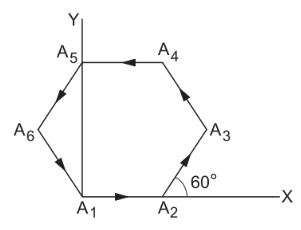Solution:

According to polygon vector addition, the resultant of these six vectors is 0.

Here magnitudes, A = B = C = D = E = F

Now,

Resultant of x component = Rx = A cos0 + A cosπ/3 + A cos2π/3 + A cos3π/3 + A cos4π/3 + A cos5π/3 = 0

[component of resultant is zero as resultant is zero]

=> cos0 + cosπ/3 + cos2π/3 + cos3π/3 + cos4π/3 + cos5π/3 = 0

Similarly, it can be proved that sin0 + sinπ/3 + sin2π/3 + sin3π/3 + sin4π/3 + sin5π/3 = 0

Question 13: Let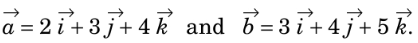Find the angle between them.

Solution:

Dot product of vector a and vector b.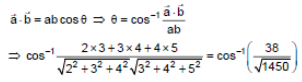Question 14: Prove that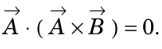Solution: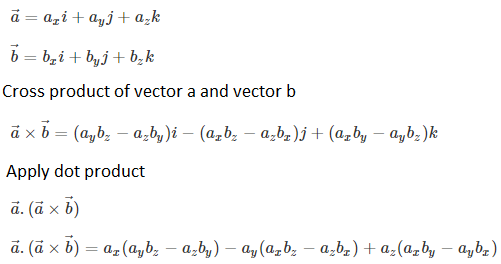Question 15: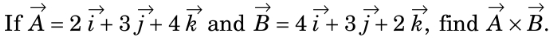Solution: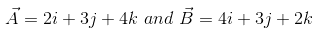Find Cross product of given vectors: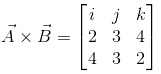= i(6-12) – j(4-16) + k(6-12)

= -6i + 12j – 6k

Question 16: If vectors A, B, C are mutually perpendicular, show that

Is the converse true?

Solution:

Given: A , B , C are mutually perpendicular vectors.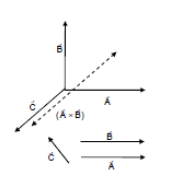Vector A and B is a vector whose direction is perpendicular to the plane containing A and B.

Also vector C is perpendicular to A and B.

Angle between vector C and A x B is 180° or 0° .

=> $\vec C \times (\vec A \times \vec B)=0$

But, the converse is not true.

Because, If two vector are parallel then,

So , they need not be mutually perpendicular.

Question 17: A particle moves on a given straight line with a constant speed v. At a certain time it is at a point P on its straight line path. O is a fixed point. Show that is independent of the position P.

Solution: The particle moves on the straight line PP’ at speed v.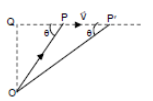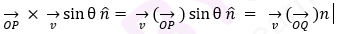and

OQ = OP sin θ = OP’ sin θ’ (from figure)

So, the magnitude of OP x v remain constant irrespective of the position of the particle.

Therefore, OP x v is independent of position P.

Question 18: The force on a charged particle due to electric and magnetic fields is given by F = qE + qv x B. Suppose E is along the X-axis and B along the Y-axis. In what direction and with what minimum speed v should a positively charged particle be sent so that the net force on it is zero?

Solution:

Given: F= qE + q(v x B) = 0

=> E = -(v x B)

image

From figure, direction of v x B should be opposite to the direction of E. Vector v should be in positive yz plane.

Now,

E = vB sinθ

or v = E / (B sinθ)

For v  to be minimum, we have θ = 90°  and v_(min) = F/B

We can conclude that the particle must be projected at a minimum speed of E/B along +ve z-axis in which θ=90°, so that force is zero.

Question 19: Given an example for which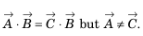Solution: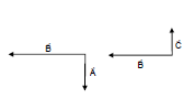From figure,

Vector A perpendicular to the vector B and Vector B perpendicular to the vector C

[Here Vector A along south side, B along west side, and vector C along north]

So dot product of vector A and B is zero => A.B=0

Therefore, A.B = B.C

or B.C = 0

But B not equal to C.

Question 20: Draw a graph from the following data. Draw tangents at x = 2, 4, 6 and 8. Find the slopes pf these tangents. Verify that the curve drawn is y = 2x2 and the slope of tangent is tan θ = dy/dx = 4x.

 x 1 2 3 4 5 6 7 8 9 10 y 2 8 18 32 50 72 98 128 162 200

Solution:

The graph of y = 2x2

Draw a tangent at the point and extend the line to cut x-axis to find the slope at any point.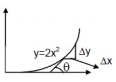from the figure,

Slope = tan θ = dy/dx = d/dy (2x2) = 4x

where x is the x-coordinate of the point where the slope is to be measured.

Question 21: A curve is represented by y = sin x. If x is changed from π/3 to π/3 + π/100 , find approximately the change in y.

Solution:

Given equation of curve is y = sin x

Change in variables, we have

y + Δy = sin (x + Δx)

or Δy = sin (x + Δx) – sin x

= ( π/3 + π/100) – sin(π/3)

= 0.0157

Question 22: The electric current in a charging R-C circuit is given by

i = io e-t/RC

where io, R and C are constant parameters of the circuit and t is time. Find the rate of change of current at (a) t = 0, (b) t = RC, (c) t = 10 RC.

Solution:

Given:

i = i₀e(-t/RC)

Find rate of change of current:

Differentiate I w.r.t. t, we get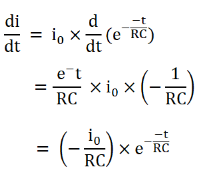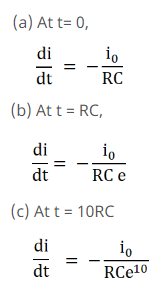Question 23: The electric current in a discharging R-C circuit is given by i = io e-t/RC

where io, R and C are constant parameters and t is time. Let i0 = 2.00 A, R = 6.00 × 105 Ω and C = 0.500 μF. (a) Find the current at t = 0.3 s

(b) Find the rate of change of current at t = 0.3 s

(c) Find approximately the current at t = 0.31 s.

Solution:

Given ; i = 2 A,

R =6 x 10⁵ Ω

and C = 0.500 μF or 5 x10⁻⁷ F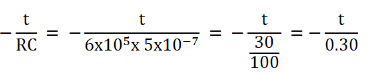Now,

The electric current in a discharging R-C circuit is, i = 2 e-t/0.30 …(1)

a) Current at t = 0.3 s

(1) => i = 2 × e-1 = (2/e) A.

(b) Rate of change of current at t = 0.3 s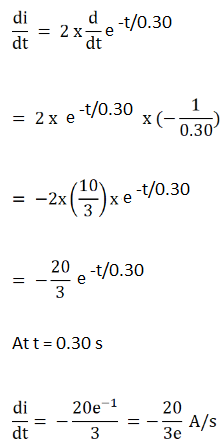(c) Approximately the current at t = 0.31 s.

At t = 0.31 sec, i = 2e(-0.3/0.3) = (5.8/3e) A

Question 24: Find the area bounded under the curve y = 3×2 + 6x + 7 and the X-axis with the ordinates at x = 5 and x = 10.

Solution:

Let P(x, y) be a point on the curve between x=5 and x=10 and dx is a very small increment next to x. Now the area of the small strip is dA = y dx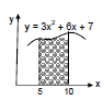Area =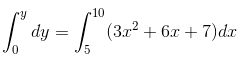= 1135 sq. units

Question 25: Find the area enclosed by the curve y = sin x and the X-axis between x = 0 and x = π.

Solution:

Equation of the curve, y = sin x.

The required area can found by integrating y w.r.t. x :

Area = ∫y dx = ∫ sin x dx

= -[cos x]0π = 2

Question 26: Find the area bounded by the curve y = e-x, the X-axis and the Y-axis.

Solution:

Equation of curve is

When x = 0, y = e−0 = 1

x increases, the value of y decrease.

Also, only when x = ∞, y = 0

So, the required area can be found by integrating the function from 0 to ∞.

Area = ∫ dA = ∫ y dx = ∫ e-x dx = e-x + C

Integration between o to ∞

= (0 + 1)

= 1

Question 27: A rod of length L is placed along the X-axis between x = 0 and x = L. The linear density (mass/length) ρ of the rod varies with the distance x from the origin as ρ = a + bx. (a) Find the SI units of a and b.

(b) Find the mass of the rod in terms of a, b and L.

Solution:

Given: Density = ρ = mass/length = a + bx

So, the SI unit of ρ is kg/m.

(a)

SI unit of a = kg/m

SI unit of b = kg/m2

[(From the principle of homogeneity of dimensions]

(b) Let us consider a small element of length dx at a distance x from the origin

image

we have,

dm = mass of the element = ρ dx = (a + bx)dx

Therefore, mass of rod = m = ∫dm = ∫(a+bx) = ax + bx2/2

[Integration between o to L]

= aL + bL2/2

Question 28: The momentum p of a particle changes with time t according to the relation dp/dt = (10N) + (2N/s)t. If the momentum is zero at t = 0, what will the momentum be at t = 10 s?

Solution:

The momentum at time t

p = ∫ dp = ∫ [(10N) + (2N/s)t] dt

= (10 t + t2) + C N/s

Where C = constant of integration

Given at t = 0, p = 0.

So, above equation is turn as

0 = C

or C = 0

=> p = (10 x 100 + 102) N/s = 200 Ns or 200 kg m/s

Question 29: The changes in a function y and the independent variable x are related as dy/dx = x2. Find y as a function of x.

Solution:

Given: The changes in a function y and the independent variable x are related as dy/dx = x2.

=> dy = x2 dx

By integrating both sides,we get

∫dy = ∫ x2 dx

or y = x3/3 + c ; c= constant of integration

Therefore, y as a function of x is represented by x3/3 + c

Question 30: Write the number of significant digits’ in

(a) 1001

(b) 100.1

(c) 100.10

(d) 0.001001

Solution:

(a)1001

Number of significant digits = 4

(b) 100.1

Number of significant digits = 4

(c) 100.10

Number of significant digits = 5

(d) 0.001001

Number of significant digits = 4

Question 31: A meter scale is graduated at every millimeter. How many significant digits will be there in a length measurement with this scale?

Solution: We know, 1 m = 1000 mm

The minimum number of significant digits can be 1 (for example, for measurements like 2 mm and 6 mm) and the maximum number of significant digits can be 4 (for example, for measurements like 1000 mm).

The number of significant digits may be 1, 2, 3 or 4.

Question 32: Round the following numbers to 2 significant

(a) 3472 (b) 84.16 (c) 2.55 and (d) 28.5

Solution:

(a) In Value 3472, after digit 4, 7 is there which is greater than 5.

So, neglect next two digits and the values of 4 is increased by 1.

Value is 3500

(b) 84.16

Required number is 84

(c) 2.55

Required number is 2.6

(d) 28.5

Required number is 28.

Question 33: The length and the radius of a cylinder measured with a slide calipers are found to be 4.54 cm and 1.75 cm respectively. Calculate the volume of the cylinder.

Solution:

From given information’s,

Length of the cylinder, l = 4.54 cm

Radius of the cylinder, r = 1.75 cm

Now,

Volume, V = πr2l = π (1.75) 2 (4.54)

= 3.14 x 1.75 x 1.75 x 4.54

= 43.6577

The minimum number of significant digits in a particular term is three. Therefore, rounded off the result into three significant digits, we have:

V = 43.7 cm3

Question 34: The thickness of a glass plate is measured 2.17 mm, 2.17 mm and 2.18 mm at three different places. Find the average thickness of the plate from this data.

Solution:

Given: The thickness of a glass plate is measured 2.17 mm, 2.17 mm and 2.18 mm at three different places.

Average thickness = (2.17+2.17+2.18)/3 mm = 2.1733 mm

Round off above result to three significant digits, the average thickness becomes 2.17 mm

Question 35: The length of the string of a simple pendulum is measured with a meter scale to be 90.0 cm. The radius of the bob plus the length of the hook is calculated to be 2.13 cm using measurements with a slide calipers. What is the effective length of the pendulum? (The effective length is defined as the distance between the point of suspension and the center of the bob.)

Solution: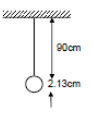Given:

The length of the simple pendulum = 90 cm and

The radius of bob and hook = 2.13 cm

From figure,

Actual effective length of the pendulum = (90.0 + 2.13) cm

However, in the measurement 90.0 cm, the number of significant digits is only 2.

So, the effective length should contain only two significant digits.

Therefore, the effective length of the pendulum = 90.0 + 2.13 = 92.1 cm.